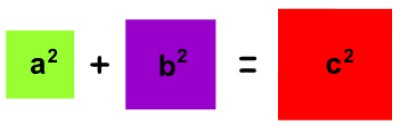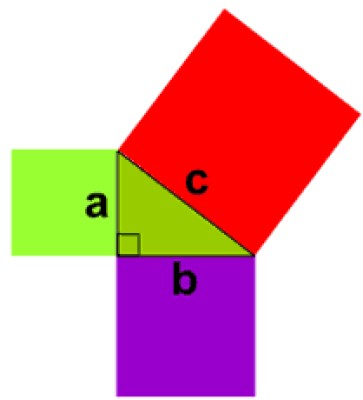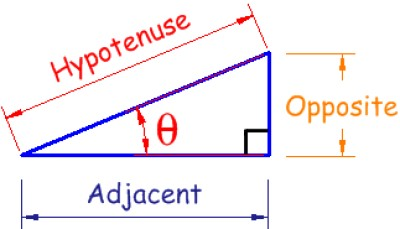List of public pages created with Protopage

# Notes

## Plain sticky notes

### Sticky note

Click this text to change it...

# Unit A

## Photos

### Pythagoras' Theorem

After you find what C squared equals, you will need to square root it to find the missing length.# Unit C

## Plain sticky notes

### Standard Index Form

Standard index form is also known as standard form. It is very useful when writing very big or very small numbers. In standard form, a number is always written as: A × 10 n A is always between 1 and 10. n tells us how many places to move the decimal point.

### Trigonometry

The triangle will show the lengths of different sides. Different sides mean different formulas. Opposite/Hypotenuse = Sin Adjacent/Hypotenuse = Cos Opposite/Adjacent = Tan You will find the three formulae on a scientific calculator.

### Standard Index Form Example

Example: Write 15 000 000 in standard index form. Solution: 15 000 000 = 1.5 × 10 000 000 This can be rewritten as: 1.5 × 10 × 10 × 10 × 10 × 10 × 10 × 10 = 1. 5 × 10 7

## Photos

### Trigonometry